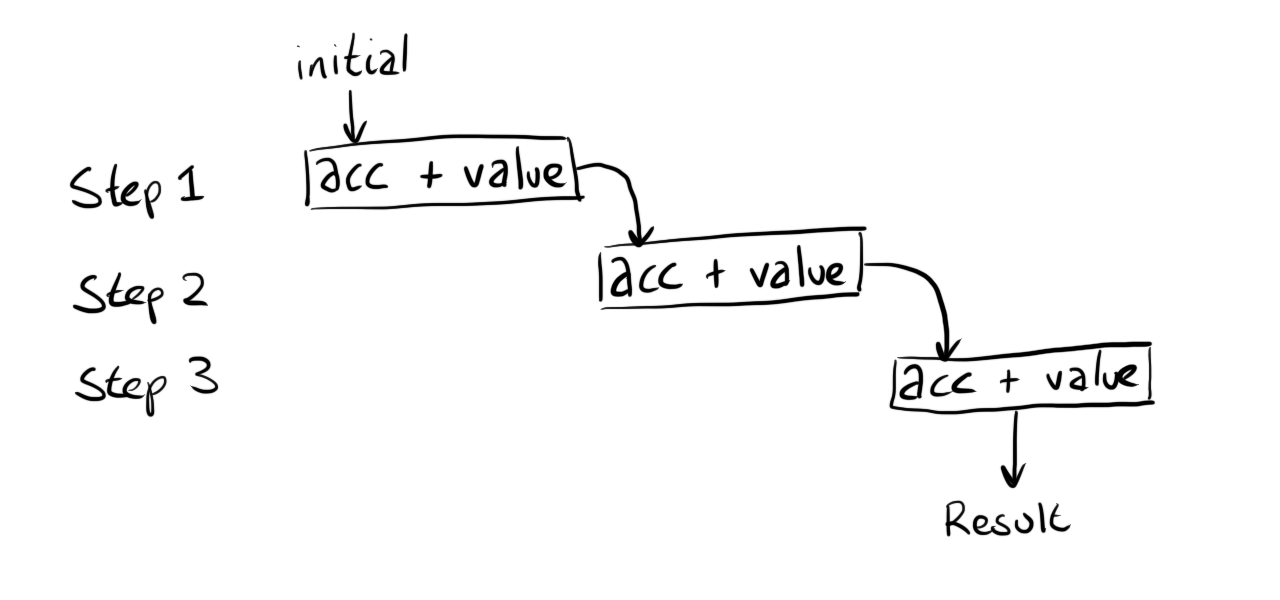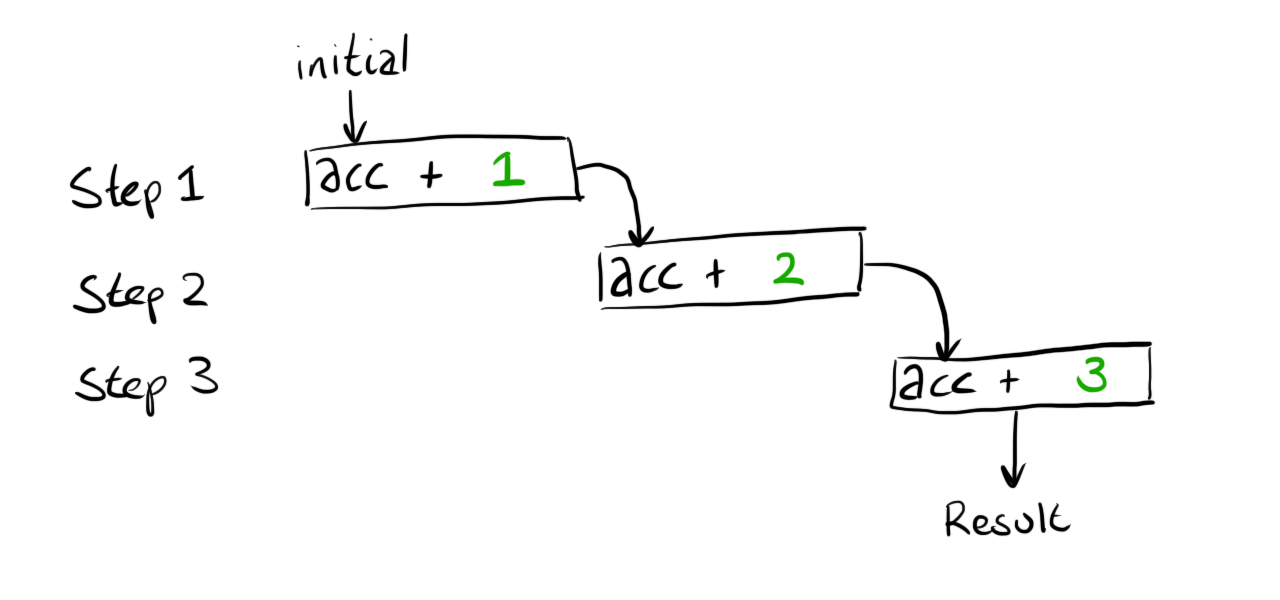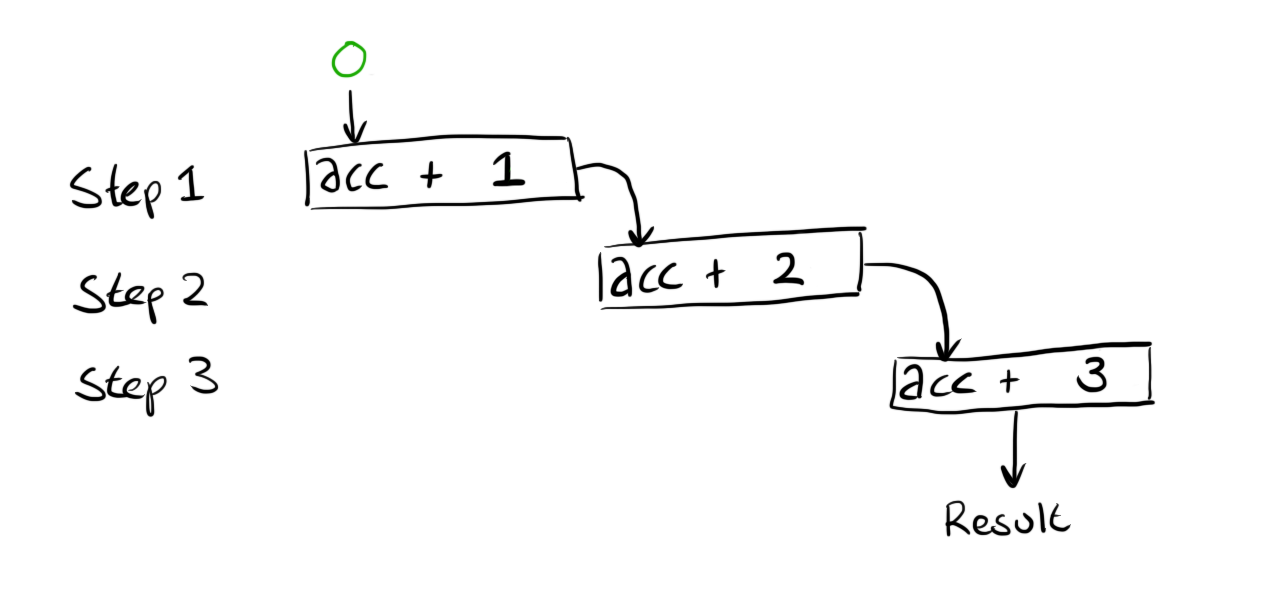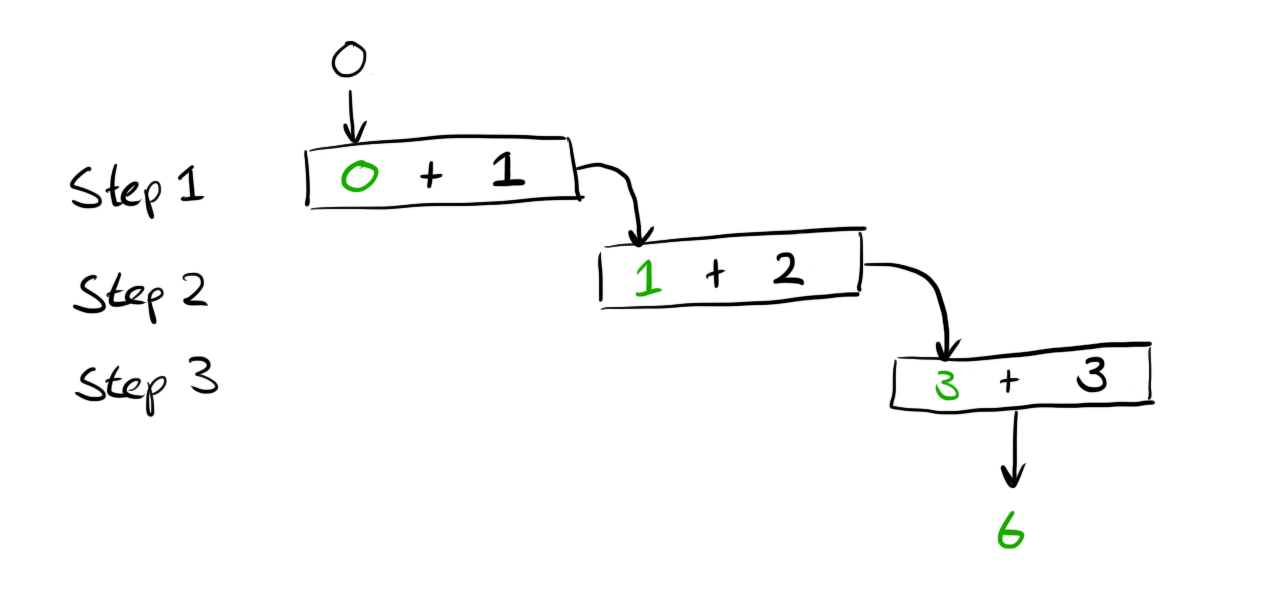# More unit testing with Excel Lambdas

For some reason I decided it would be fun to attempt Day 1 of the Advent of Code 2021 using Excel’s Lambdas, whilst unit testing as I go - here’s how that went.

The challenge can be summarised as - go through a list of values and keep track how many times the latest value is larger than the value before it. For example given this list:

``````199
200
208
210
200
207
240
269
260
263
``````

We can step through the values observing whether the value increased or decreased

``````199 (N/A - no previous measurement)
200 (increased)
208 (increased)
210 (increased)
200 (decreased)
207 (increased)
240 (increased)
269 (increased)
260 (decreased)
263 (increased)
``````

Our task is to tally how many times the value increased - in the above that would be 7.

## Reduce a list to a single value

In order to perform the calculation I’m going to need to use Excel’s `REDUCE` function. `REDUCE` takes an initial value, the range we want to enumerate and a LAMBDA that is used to combine the values together.

A small worked example might help

``````=REDUCE(0, {1,2,3}, LAMBDA(acc, value, acc + value))
``````

The expression above will evaluate to 6 but let’s try and visualise what is happening. We have 3 items in our array which means that the combining function is going to be called 3 times - I can show this with some boxes representing each step:If we substitute the values that were passed to the function (`{1,2,3}`) the diagram looks like this:We can’t compute the result because the first calculation still needs a value for `acc`. This value is provided as the first argument to our call to `REDUCE` which gives this:Finally we can perform all the calculations and get the final result of `6`.The above example is obviously not something we’d want to ever use, especially as it’s a long winded way of writing `=SUM(1,2,3)` but it shows how `REDUCE` is performing all the iteration for us and we just have to provide a combining function to tailor the behaviour.

## Working around single value returns

Unfortunately `REDUCE` alone won’t quite get us what we need. When we iterate over the list we actually need to keep track of two pieces of data, the current count of increments and the previous value we’ve seen. As seen above `REDUCE` only keeps track of a single value in the variable we called `acc`.

The way I’m going to work around this restriction is to create a `Pair` abstraction. I’m going to create a few helper functions that work on arrays with the expectation that the array only ever has 2 elements.

I’ll start by writing some tests for these functions in a new sheet called `PairTests`.

A (Formula) A (Result) B C (Formula) C (Result)
1 Actual Actual Expected Result Result
2 `=ARRAYTOTEXT(PairMake(0,0))` 0, 0 0, 0 `=Assert.Equals(B2,C2)`
3 `=ARRAYTOTEXT(PairMake(0,1))` 0, 1 0, 1 `=Assert.Equals(B3,C3)`
4 `=ARRAYTOTEXT(PairMake(1,1))` 1, 1 1, 1 `=Assert.Equals(B4,C4)`

The above tests are verifying that a function called `PairMake` can create arrays correctly.
*I’m using `ARRAYTOTEXT` to make the assertion a little easier to write
**Naming is hard and `PairMake` is the best I could come up `¯\_(ツ)_/¯`

To make this test pass I can write the formula as

``````PairMake = lambda(x,y,MAKEARRAY(1,2,lambda(_, col,IF(col=1,x,y))));
``````

This function mainly exists to hide all the ugly `MAKEARRAY` stuff - there may be much easier ways to create an array with dynamic input but my googlefu was not working and I wanted to move on.

The `Assert.Equals` function I’m calling is defined like this:

``````Assert.Equals = LAMBDA(actual, expected, if(actual=expected, "", "Expected '" & expected & "' but got '" & actual & "'"));
``````

This does the job but because there is no output on success it is not obvious if anything is happening. Luckily I’ve just created a `Pair` type so I can improve the assert function by returning pairs where the first value is a pretty emoji ✅ or ❌ and the second value is empty in the success case or the helpful error message in the failure case.

``````Assert.Equals = LAMBDA(actual, expected, if(actual=expected, PairMake("✅",""), PairMake("❌","Expected '" & expected & "' but got '" & actual & "'")));
``````

After refreshing the previous table now looks healthier and shows some calculation is actually being done

A (Formula) A (Result) B C (Formula) C (Result)
1 Actual Actual Expected Result Result
2 =ARRAYTOTEXT(PairMake(0,0)) 0, 0 0, 0 =Assert.Equals(B2,C2)
3 =ARRAYTOTEXT(PairMake(0,1)) 0, 1 0, 1 =Assert.Equals(B3,C3)
4 =ARRAYTOTEXT(PairMake(1,1)) 1, 1 1, 1 =Assert.Equals(B4,C4)

Next up I want to define two more functions to complete the illusion that I have a `Pair` and hide the fact that I’m just using an Array.

A (Formula) A (Result) B C (Formula) C (Result)
1 Actual Actual Expected Result Result
2 =Pair.First({“first”,”second”}) first first =Assert.Equals(B2,C2)
3 =Pair.Second({“first”,”second”}) second second =Assert.Equals(B3,C3)

Having functions to grab both the elements from the `Pair` means I don’t need to mess with `INDEX` everywhere and ruin the illusion that I have an honest `Pair` type. Under the hood these functions do in fact just use `INDEX`

``````Pair.First = lambda(pair,INDEX(pair,1));
Pair.Second = lambda(pair,INDEX(pair,2));
``````

## Actually solving the challenge

The above was a lot of scaffolding/understanding that means we are now able to get to the task at hand. As a recap we are going to use `REDUCE` to iterate over some data, whilst iterating we are going to keep track of the current count of increases and the previous value we are working with. Once the iteration is done we can discard the previous value and just pluck out the count we have been accumulating.

Assuming the data is contained in a sheet named `Data` let’s see how this all looks plumbed together

``````=Pair.First(
REDUCE(
PairMake(0,Data!A1),
Data!A1:Data!A10,
??????
)
)
``````

Our reduce is going to produce a `Pair` where the first element is the count and the second element is the previous value. We don’t care about the previous element at the end of our calculation so we just pluck out the first value by wrapping the `REDUCE` in a call to `Pair.First`.

The first argument to `REDUCE` is the initial value - above we are creating a `Pair` where the first element represents the count starting at `0`. The second element represents the previous value, which we are setting to the first value in the list. This is because if you look at the problem example at the top of this post the first item does not count as an increment as there was no previous value.

The second argument to `REDUCE` is the data range - nothing to explain here.

The third argument is MIA. I’ve jumped ahead too much and we probably need to figure out the behaviour of this combining function by writing some tests.

## The combining function

The combining function will be called for each element in the array. Each time we will have available the count of increments + the previous value wrapped in a pair and the current value. The combining function needs to figure out if the increasing count needs incrementing and then it always needs to return the current value. These two pieces of information are then fed into the next round of calculation and so on until we reach the end of our collection.

We can express these requirements with 3 tests:

A (Formula) A (Result) B C (Formula) C (Result)
1 Actual Actual Expected Result Result
2 =ARRAYTOTEXT(NextPartialResult({0,0},1)) 1, 1 1, 1 =Assert.Equals(B2,C2)
3 =ARRAYTOTEXT(NextPartialResult({0,1},1)) 0, 1 0, 1 =Assert.Equals(B3,C3)
4 =ARRAYTOTEXT(NextPartialResult({1,2},1)) 1, 1 1, 1 =Assert.Equals(B4,C4)

The test in Row 2 checks that the count is incremented when the current value is bigger than the previous.
The test in Row 3 checks that the count is not incremented when the current value is equal to the previous.
The test in Row 4 checks that the count is not incremented when the current value is less than the previous.

A function that satisfies these tests could look like this

``````NextPartialResult = LAMBDA(acc, value,
Let(
count, Pair.First(acc),
previous, Pair.Second(acc),
if(
previous<value,
PairMake(count+1,value),
PairMake(count,value)
)
)
);
``````

## Plumbing it all together

With all the above we can now complete the task by filling out the `??????` in our previous attempt

``````=Pair.First(
REDUCE(
PairMake(0,Data!A1),
Data!A1:Data!A10,
NextPartialResult
)
)
``````

We can now sit back and marvel at the glowing number `7` that we could have calculated by hand on such a small data set with considerably less effort. Thankfully the actual challenge has a data set with 2000 lines and seeing the work above calculate correctly against the full data set is much more rewarding.

If you got this far and you are thinking that you could solve the problem in a simpler way using a different formula then check out the next post where I do exactly that but in a controlled way using tests to ensure a completely different formula behaves identically.